# How to Find the Foci of Ellipses & Hyperbolas

Cynthia Helzner, Laura Pennington
• Author
Cynthia Helzner

Cynthia Helzner has tutored middle school through college-level math and science for over 20 years. She has a B.S. in microbiology from The Schreyer Honors College at Penn State and a J.D. from the Dickinson School of Law. She also taught math and test prep classes and volunteered as a MathCounts assistant coach.

• Instructor
Laura Pennington

Laura received her Master's degree in Pure Mathematics from Michigan State University, and her Bachelor's degree in Mathematics from Grand Valley State University. She has 20 years of experience teaching collegiate mathematics at various institutions.

Learn about the foci of hyperbola and the foci of an ellipse. See a comparison of an ellipse vs. a hyperbola. Find out what the foci of an ellipse are using an example. Discover how to find foci of hyperbola. Updated: 02/09/2022

Show

## Ellipse vs. Hyperbola

Circles, ellipses, parabolas, and hyperbolas are known collectively as conic sections because they can be formed by slicing a cone. Conic sections were discovered by the ancient Greek mathematician Menaechmus in approximately 360-350 B.C.E. Aristaeus, Euclid, and Archimedes further contributed to the study of conic sections. All conic sections have either one or two foci.

A focal point, or focus, (plural: foci) is a point at which waves bouncing off of a curve will collect. For example, the antenna in a satellite dish is the focus of the dish because all signals hitting the bowl of the dish will bounce off of the dish and hit the antenna, thus focusing the signal at that point. Ellipses and hyperbolas have two foci while circles and parabolas (on which satellite dishes are based) have only one focus. Ellipses and hyperbolas have very similar equations and the quickest way to identify an ellipse vs. hyperbola equation is by the plus or minus sign between the two fractions: ellipses have a plus sign while hyperbolas have a minus sign.

### What is an ellipse?

An ellipse is the set of points such that the sum of the focal point to ellipse distances is constant. In other words, the sum of the distance from one focal point, F, to any given point, P, on the ellipse and the distance from the other focal point, G, to point P is constant: FP + GP = constant.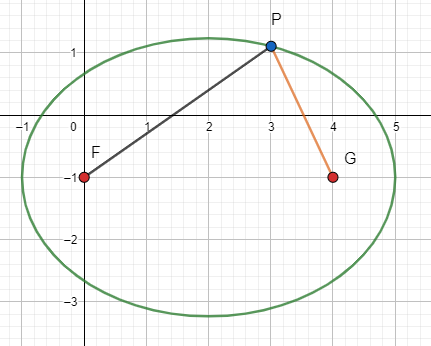Ellipses have two vertices (singular: vertex), which are the endpoints of the major axis, i.e. the longest diameter of the ellipse. They also have two covertices (singular: covertex), which are the endpoints of the minor axis, which is perpendicular to the major axis. The equation of a horizontal ellipse, i.e. an ellipse that is wider than it is tall, is {eq}\frac{(x-h)^2}{a^2} + \frac{(y-k)^2}{b^2} = 1 {/eq} where (h, k) is the center, a is the major radius, which is half of the major axis, and b is the minor radius, which is half of the minor axis. The equation of a vertical ellipse, i.e. an ellipse that is taller than it is wide, is {eq}\frac{(x-h)^2}{b^2} + \frac{(y-k)^2}{a^2} = 1 {/eq} where (h, k) is the center, a is the major radius, and b is the minor radius.

A real-life application of an ellipse and its foci occurs in a whispering gallery. Its ellipsoid shape allows a whisper at one focus to be heard at the other focus even though a whisper is too quiet to hear elsewhere in the gallery.

### What is a hyperbola?

A hyperbola is the set of points such that the absolute value of the difference between the focal point to hyperbola distances is constant. In other words, the positive difference between the distance from one focal point, F, to any given point, P, on the hyperbola and the distance from the other focal point, G, to point P is constant: |FP - GP| = constant.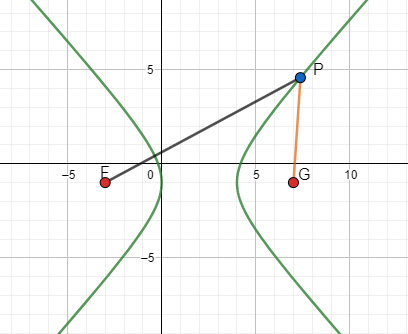Hyperbolas look roughly like a set of two parabolas and they thus have two vertices, which are the roughly U-shaped or V-shaped corners of the hyperbola. The equation of a horizontal hyperbola, i.e. a hyperbola that opens left and right, is {eq}\frac{(x-h)^2}{a^2} - \frac{(y-k)^2}{b^2} = 1 {/eq} where (h, k) is the center, a is the distance from the center to a vertex, and b is the positive asymptote slope multiplied by a. The equation of a vertical hyperbola, i.e. a hyperbola that opens up and down, is {eq}\frac{(y-k)^2}{a^2} - \frac{(x-h)^2}{b^2} = 1 {/eq} where (h, k) is the center, a is the distance from the center to a vertex, and b is the reciprocal of the positive asymptote slope divided by a.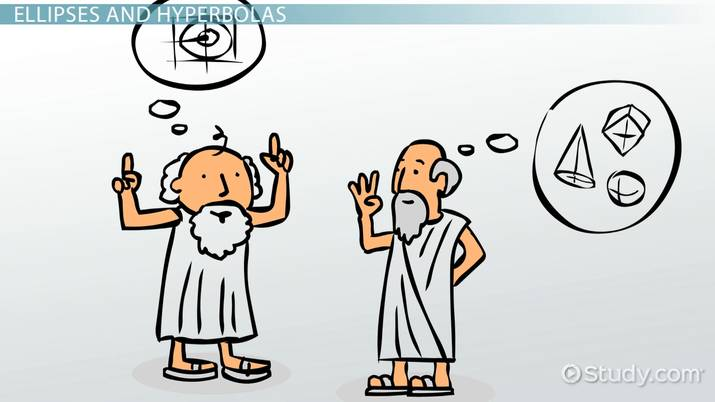An error occurred trying to load this video.

Try refreshing the page, or contact customer support.

Coming up next: Derive the Equation of an Ellipse from the Foci

### You're on a roll. Keep up the good work!

Replay
Your next lesson will play in 10 seconds
• 0:02 Ellipses and Hyperbolas
• 1:10 Foci of an Ellipse and…
• 2:46 Some Examples
• 5:02 Lesson Summary
Save Save

Want to watch this again later?

Timeline
Autoplay
Autoplay
Speed Speed

## Foci of Ellipses

The foci of an ellipse are the two points at which waves bouncing within the ellipse will collect. One can draw the ellipse by knowing its foci and the measurement of the major or minor radius because the focal distance, c, (the distance from the center to either focus, or half of the distance between the two foci) is related to the major radius, a, and the minor radius, b, by the equation {eq}a^2 - b^2 = c^2 {/eq}. Thus, knowledge of any two of those quantities is sufficient to calculate the third.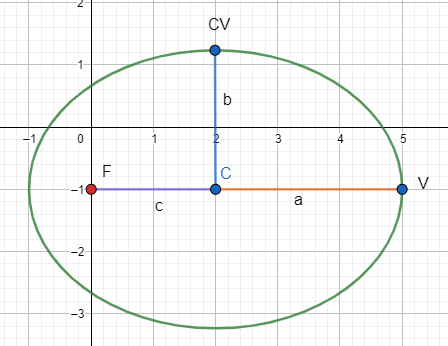Likewise, one can find the foci by knowing the center point, which is the midpoint of the vertices, the type of ellipse (vertical or horizontal), and the focal distance. The foci are equidistant from the center point and are located along the major axis, which will be horizontal for a horizontal ellipse and vertical for a vertical ellipse. For example, in a horizontal ellipse with center point is (2, -1) and focal distance 2, the foci are each 2 units away from the center point. It is a horizontal ellipse so move 2 units left and 2 units right from the center point to locate the foci: (0, -1) and (4, -1).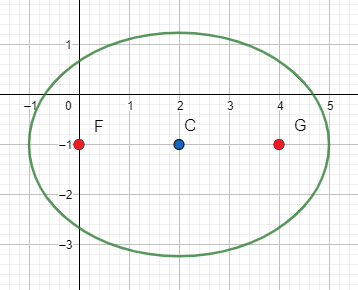### Example 1: What are the Foci of an Ellipse?

What are the foci of an ellipse with vertices and covertices at (0, 2), (10, 2), (5, 5), and (-1, 5)?

First, plot the four points (sketching the ellipse is optional for this question) and find the midpoint of the vertices, the midpoint of the covertices, or draw the major and minor axes to see where they cross. Any of those three methods gives the center point, C: (5, 2).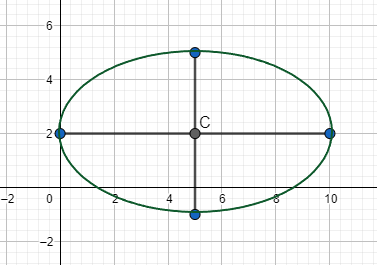Next, find the major radius, a, by finding the distance between point C and either vertex. That distance is 5 so a = 5. Then, find the minor radius, b, by finding the distance between point C and either covertex. That distance is 3 so b = 3. Since {eq}a^2 - b^2 = c^2 {/eq}, plugging in the values of a and b yields {eq}5^2 - 3^2 = c^2 {/eq} so {eq}16 = c^2 {/eq}. Therefore, c = 4 so the foci are each 4 units away from point C.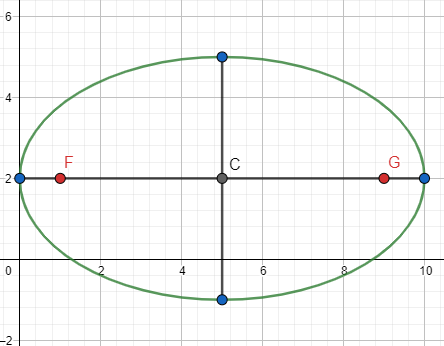To unlock this lesson you must be a Study.com Member.

#### What is the formula of a hyperbola?

The equation of a hyperbola depends on whether it is horizontal or vertical. The equation of a horizontal hyperbola is (x-h)^2/a^2 - (y-k)^2/b^2 = 1, while the equation of a vertical hyperbola is (y-k)^2/a^2 - (x-h)^2/b^2 = 1.

#### Does a hyperbola have two foci?

Yes, a hyperbola has exactly two foci. In fact, the definition of a hyperbola requires the hyperbola to have two foci. A hyperbola is defined as the set of points such that the positive difference between the following two quantities is constant: (1) the distance from one focal point to any given point, P, on the hyperbola, and (2) the distance from the other focal point to point P.

#### How do you find the foci of an ellipse?

Subtract the smaller denominator in the equation of the ellipse from the larger one, then square root the result to find the focal distance, c. For a horizontal ellipse, move c units left and right from the center to locate the foci. For a vertical ellipse, move c units up and down from the center to locate the foci.

#### What is the foci of a hyperbola?

Add the two denominators in the equation of the hyperbola, then square root the result to find the focal distance, c. For a horizontal hyperbola, move c units west and east from the center to locate the foci. For a vertical hyperbola, move c units north and south from the center to locate the foci.

### Register to view this lesson

Are you a student or a teacher?